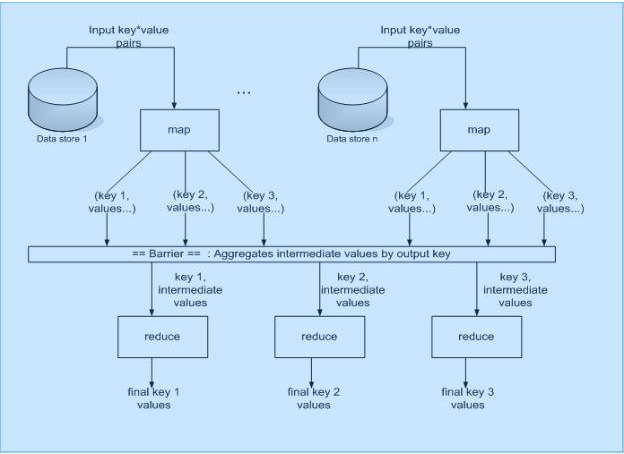Rating: 4

6864
1. Share:

## MapReduce Programming Model

Google’s MAPREDUCE IS A PROGRAMMING MODEL serves for processing large data sets in a massively parallel manner. We deliver the first rigorous description of the model, including its advancement as Google’s domain-specific language Sawzall. To this end, we reverse-engineer the seminal papers on MapReduce and Sawzall, and we capture our findings as an executable specification. We also identify and resolve some obscurities in the informal presentation given in the seminal papers. We use typed functional programming (specifically Haskell) as a tool for design recovery and executable specification. Our development comprises three components:

(i) the basic program skeleton that underlies MapReduce computations;
(ii) the opportunities for parallelism in executing MapReduce computations;
(iii) the fundamental characteristics of Sawzall’s aggregators as an advancement of the MapReduce approach.

Enthusiastic about exploring the skill set of MapReduce? Then, have a look at the MAPREDUCE TRAINING together additional knowledge.

Our development does not formalize the more implementational aspects of an actual, distributed execution of MapReduce computations.

The MapReduce programming model is straightforward, and borrows from the simplicity of functional programming. In the MapReduce programming model, the developer expresses the computation goal as the implementation of two primitive functions: map() and reduce(). The programming model for MapReduce is often expressed as follows:

map (k1, v1)                      ->                 list(k2, v2)

reduce (k2, list(v2))         ->                 list(v2)

In the above model, the map() function is run in parallel against an input list of key(k1) value(v1) pairs. The map() implementation can perform any type of computation, the software engineer wishes, with the one caveat that the output of the procedure is a list of key*value pairs as well: key(k2) and intermediate value(v2) as shown in the model above. The MapReduce implementation performs the shuffling of the output list into the appropriate reduce() functions so that logically the reduce() function processes the same key(k2) and intermediate value(v2). Thus the reduce() function does not have to keep track of different keys. The reduce() function, with the k2, v2 input, is able to perform a number of processes such as aggregation, sort, transformation, etc. While MapReduce is not suited to some tasks, the programming model is ideal for situations where analysis must be performed on a large amount of distributed data.The figure below shows a data flow orientation of the MapReduce programming model. In the figure, one or more data stores contain a list of key*value pairs. The MapReduce implementation coordinates the invocation of these lists into a set of processes running the map() functions.

The map() function is run in parallel until the entire input key*value pairs are processed. A barrier is held to ensure the last input key*value pair is processed by map() before the reduce() function can begin. The underlying MapReduce implementation is responsible for shuffling the different intermediate keys*value to the appropriate reduce() functions based on the value of the key.Figure 2 – Map Reduce Data Flow (King)

One of the tasks MapReduce is appropriate for is counts of certain strings across large numbers of files such as logs, files, websites, etc. For example, a Map Reduce pseudo-code for determining the number of times a URL is found in a website is listed below. The map() function in this example processes a list of websites. In this example, the key is not important, but the value contains the HTML markup of the website. For each case where the HREF is found in the markup, map() emits a name*value pair of the HREF and “1’. Reduce simply adds all of the similar HREF keys together and emits a total count for each.

``````map(String key, String value){ //key
HTML Page Name //value
HTML Content for each
HREF in value
EmitIntermediate(HREF, “1”);
}
reduce(String key, Interator values){
key : a word
values: a list of counts int result = 0;
for each v in values
result +=ParseInt(v);
Emit(AsString(key+”,”+result));
}``````

Another task that MapReduce is suited extremely well for is sorting large numbers of records on distributed files. Certain implementations of MapReduce have been able to sort billions of records in a short amount of time. The implementation of a MapReduce sort routine is shown below. In the example below, the map() function extracts the key to use for sorting from the value. The barrier between map() and reduce() within the MapReduce framework shuffles the output of the map() function into the appropriate sort order. In this example, reduce() does very little other than pass through the sorted list.

``````map(String key, String value){ //key
HTML Page Name //value
HTML Content
string key = extractKeyFromValue(value); EmitIntermediate(key, value);
}
reduce(String key, Interator values){
key : a word
values: a list of counts Emit(key+” “+value);
}``````

### List of Other Big Data Courses:Course Schedule
NameDates
MapReduce TrainingFeb 07 to Feb 22
MapReduce TrainingFeb 11 to Feb 26
MapReduce TrainingFeb 14 to Mar 01
MapReduce TrainingFeb 18 to Mar 05
Last updated: 04 February 2023